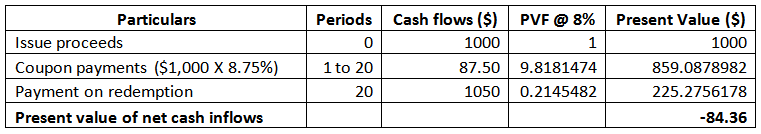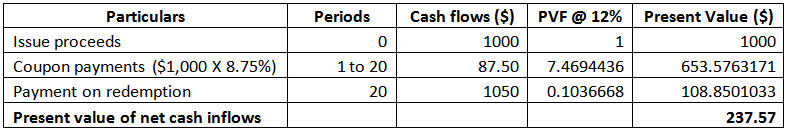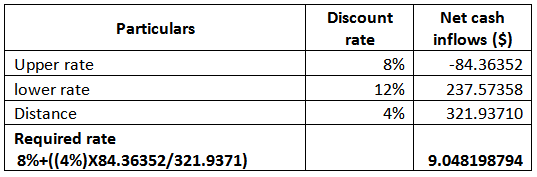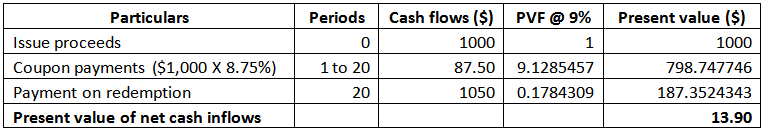# Masters Corp. issued two bonds with 20-year maturities. Both bonds are callable at \$1,050. The first bond was issued at a deep discount with a coupon rate of 4% and a price of \$580 to yield 8.4%. The second bond was issued at par value with a coupon rate of 8.75%.a. What is the yield to maturity of the par bond? Why is it higher than the yield of the discount bond?b. If you expect rates to fall substantially in the next two years, which bond has the higher expected rate of return?c. In what sense does the discount bond offer “implicit call protection”?

Question
4 views

Masters Corp. issued two bonds with 20-year maturities. Both bonds are callable at \$1,050. The first bond was issued at a deep discount with a coupon rate of 4% and a price of \$580 to yield 8.4%. The second bond was issued at par value with a coupon rate of 8.75%.
a. What is the yield to maturity of the par bond? Why is it higher than the yield of the discount bond?
b. If you expect rates to fall substantially in the next two years, which bond has the higher expected rate of return?
c. In what sense does the discount bond offer “implicit call protection”?

check_circle

Introduction

Yield to maturity (YTM) is the return provided by any investment or project if that project or investment is held till maturity. YTM is a discount rate at which present value of cash inflows is equal to the present value of cash outflows.

Bonds are financial instruments and an organization can issue bonds for raising loan. Deep discount bonds are issued at a discount and includes lower rate of interest.

Yield to Maturity

YTM can be calculated by using two discount rates; such that, the rate at which present value of net inflows is zero could fall between the two rates.

Computation of present value of net inflows using discount rate of 8%:Computation of present value of net inflows using discount rate of 12%:Interpolation of the net cash inflows to find YTM:Computation of present value of net inflows using YTM of 9%:Since, at 9% discount rate present value of net inflows is close to zero. Thus, YTM of the bond which was issued at par value is 9%.

While comparing the two bonds, redemption value of bonds is same. The issue price (net outflow) is higher in case of the par bond. Thus, higher yield is caused due to higher coupon payments received under par bonds.

Fall in Market Interest Rate

In case after the bonds are issued, interest rates in the market fell. Then in such case, investors would expect a comparatively lower rate of return.

The investor...

### Want to see the full answer?

See Solution

#### Want to see this answer and more?

Solutions are written by subject experts who are available 24/7. Questions are typically answered within 1 hour.*

See Solution
*Response times may vary by subject and question.
Tagged in